Important Questions Test: Fractions

# Important Questions Test: Fractions

Test Description

## 20 Questions MCQ Test Mathematics (Maths) Class 6 | Important Questions Test: Fractions

Important Questions Test: Fractions for Class 6 2022 is part of Mathematics (Maths) Class 6 preparation. The Important Questions Test: Fractions questions and answers have been prepared according to the Class 6 exam syllabus.The Important Questions Test: Fractions MCQs are made for Class 6 2022 Exam. Find important definitions, questions, notes, meanings, examples, exercises, MCQs and online tests for Important Questions Test: Fractions below.
Solutions of Important Questions Test: Fractions questions in English are available as part of our Mathematics (Maths) Class 6 for Class 6 & Important Questions Test: Fractions solutions in Hindi for Mathematics (Maths) Class 6 course. Download more important topics, notes, lectures and mock test series for Class 6 Exam by signing up for free. Attempt Important Questions Test: Fractions | 20 questions in 20 minutes | Mock test for Class 6 preparation | Free important questions MCQ to study Mathematics (Maths) Class 6 for Class 6 Exam | Download free PDF with solutions
 1 Crore+ students have signed up on EduRev. Have you?
Important Questions Test: Fractions - Question 1

### Express as improper fractionImportant Questions Test: Fractions - Question 2

### A two-digit number is such that the product of the digits is 8. When 18 is added to the number, then the digits are reversed. The number is:

Detailed Solution for Important Questions Test: Fractions - Question 2

Solution :- Let the two numbers be x and y respectively.

According to the question :

xy = 8 _______(i)

10x + y + 18 = 10y + x

=> 10x - x + 18 = 10y - y

=> 9(x + 2) = 9y

=> x + 2 = y ______(ii)

Putting the value y in equation (i) we get,

x(x + 2) = 8

=> x² + 2x - 8 = 0

=> x² + 4x - 2x - 8 = 0

=> x(x + 4) - 2(x + 4) = 0

=> (x - 2) (x + 4) = 0

=> x = 2 or - 4

Putting the positive value of x in equation (ii),

2 + 2 = y = 4

Hence, The number is : 2 × 10 + 4 = 20 + 4 = 24

Important Questions Test: Fractions - Question 3

### What fraction of these circles have X's in them?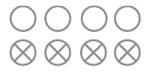Important Questions Test: Fractions - Question 4

Write the fraction representing the shaded region in the below figure.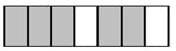Important Questions Test: Fractions - Question 5

What is the fraction of the shaded area?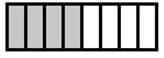Important Questions Test: Fractions - Question 6

What is the fractional form of five eighteenths?

Detailed Solution for Important Questions Test: Fractions - Question 6

The fractional form of five eighteenths

= 5/18

Important Questions Test: Fractions - Question 7

The simplest form of 16/72 is ________

Important Questions Test: Fractions - Question 8

What fraction of a day is 8 hours?

Important Questions Test: Fractions - Question 9

Express as improper fraction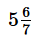Important Questions Test: Fractions - Question 10

Write the fraction representing the shaded portion.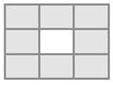Important Questions Test: Fractions - Question 11

Fractions with same denominators are called ________.

Detailed Solution for Important Questions Test: Fractions - Question 11

Like Fractions: The fractions having the same denominators are known as like fractions. We know that fractions such as 2/5, 3/5, 5/5, 7/5, 9/5, …. etc., are like fractions.

Important Questions Test: Fractions - Question 12

Express as mixed fraction 20/3.

Important Questions Test: Fractions - Question 13

The simplest form of 15/75 is ________.

Important Questions Test: Fractions - Question 14

What fraction of an hour is 40 minutes?

Important Questions Test: Fractions - Question 15

Give a proper fraction whose denominator is 7 and numerator is

Important Questions Test: Fractions - Question 16

In a proper fraction the ___________ shows the number of parts which have been considered.

Important Questions Test: Fractions - Question 17

Write the natural numbers from 102 to 113. What fraction of them are prime numbers?

Detailed Solution for Important Questions Test: Fractions - Question 17

Natural numbers from 102 to 113: 102, 103, 104, 105, 106, 107, 108, 109, 110, 111, 112, 113
Prime numbers from 102 to 113: 103, 107, 109, 113
Hence fraction of prime numbers = 4/12 = 1/3

Important Questions Test: Fractions - Question 18

A _________ means a part of a group or of a region.

Important Questions Test: Fractions - Question 19

Fractions with not same denominators are called ________.

Important Questions Test: Fractions - Question 20

Write the fraction representing the shaded region in the below figure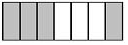## Mathematics (Maths) Class 6

228 videos|273 docs|43 tests
 Use Code STAYHOME200 and get INR 200 additional OFF Use Coupon Code
Information about Important Questions Test: Fractions Page
In this test you can find the Exam questions for Important Questions Test: Fractions solved & explained in the simplest way possible. Besides giving Questions and answers for Important Questions Test: Fractions, EduRev gives you an ample number of Online tests for practice

## Mathematics (Maths) Class 6

228 videos|273 docs|43 tests